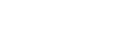## How many more accepted answers do I need until Tenacious/Unsung Hero

3

(click on this box to dismiss)

Variation of Eric's query "How unsung am I?" Zero and non-zero accepted count. Self-accepted answers do not count. How many more accepted answers with a score of zero are needed, assuming no more accepted answers with a score greater than 0, to receive both badges.Q&A for Ubuntu users and developers

``````-- How many more accepted answers do I need until Tenacious/Unsung Hero
-- Variation of Eric's query "How unsung am I?" Zero and non-zero accepted count. Self-accepted answers do not count. How many more accepted answers with a score of zero are needed, assuming no more accepted answers with a score greater than 0, to receive both badges.

select
sum(case when a.Score = 0 then 0 else 1 end) as [Scored Answers],
sum(case when a.Score = 0 then 1 else 0 end) as [Unscored Answers],
sum(CASE WHEN a.Score = 0 then 1 else 0 end)*1000 / count(a.Id) / 10.0 as [Percentage Unscored],
(count(a.Id)/5-sum(case when a.Score = 0 then 1 else 0 end))/0.8 as [To Tenacious],
(count(a.Id)/4-sum(case when a.Score = 0 then 1 else 0 end))/0.75 as [To Unsung Hero]
from
Posts q
inner join
Posts aHold tight while we fetch your results